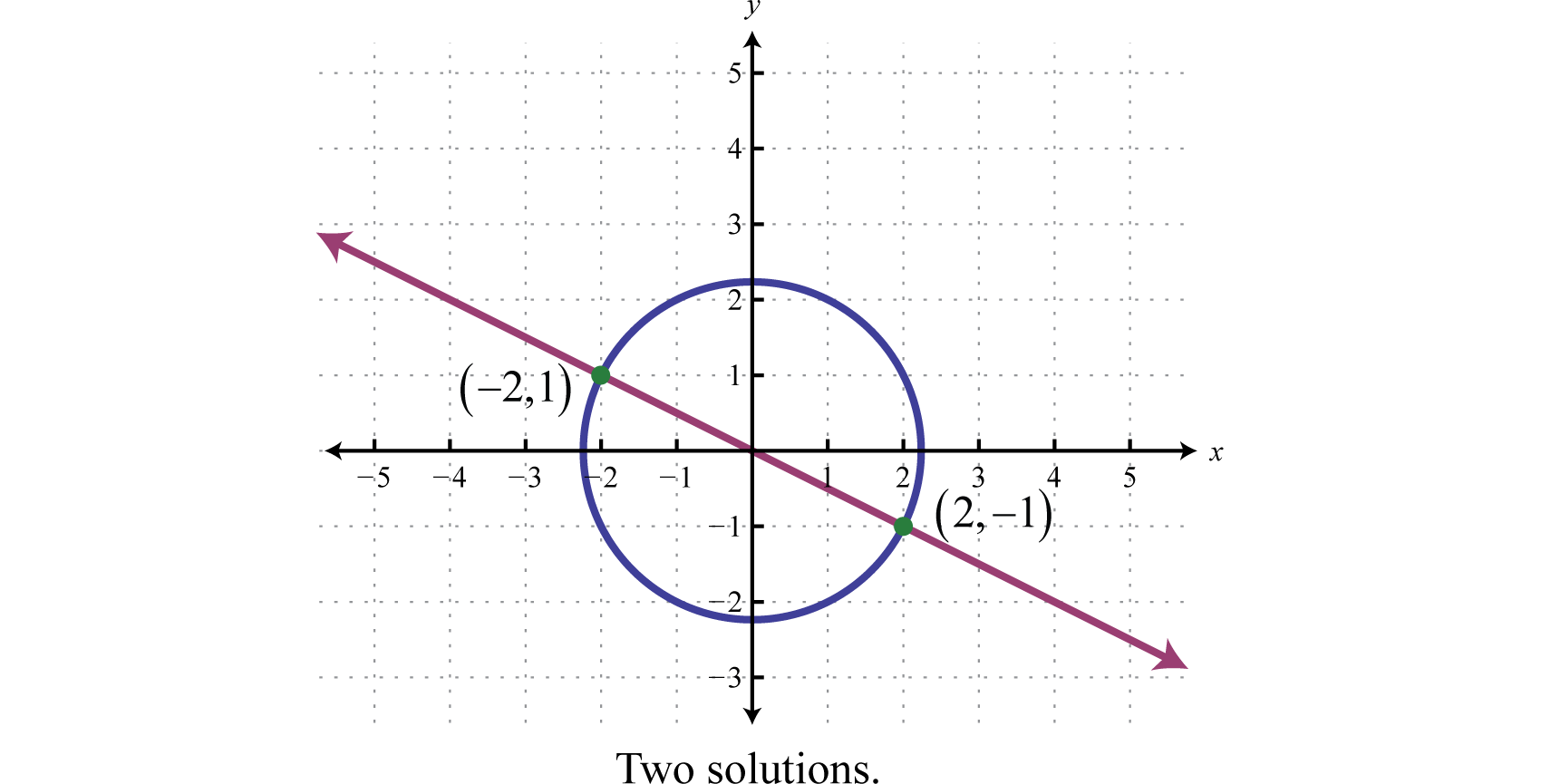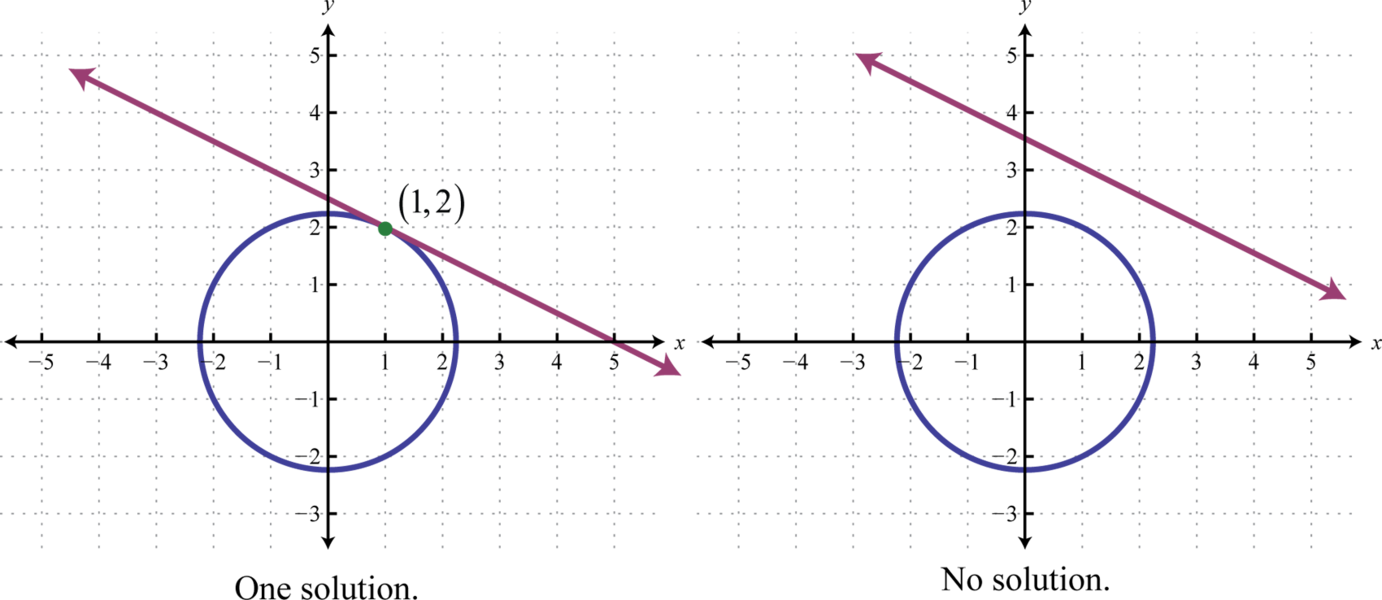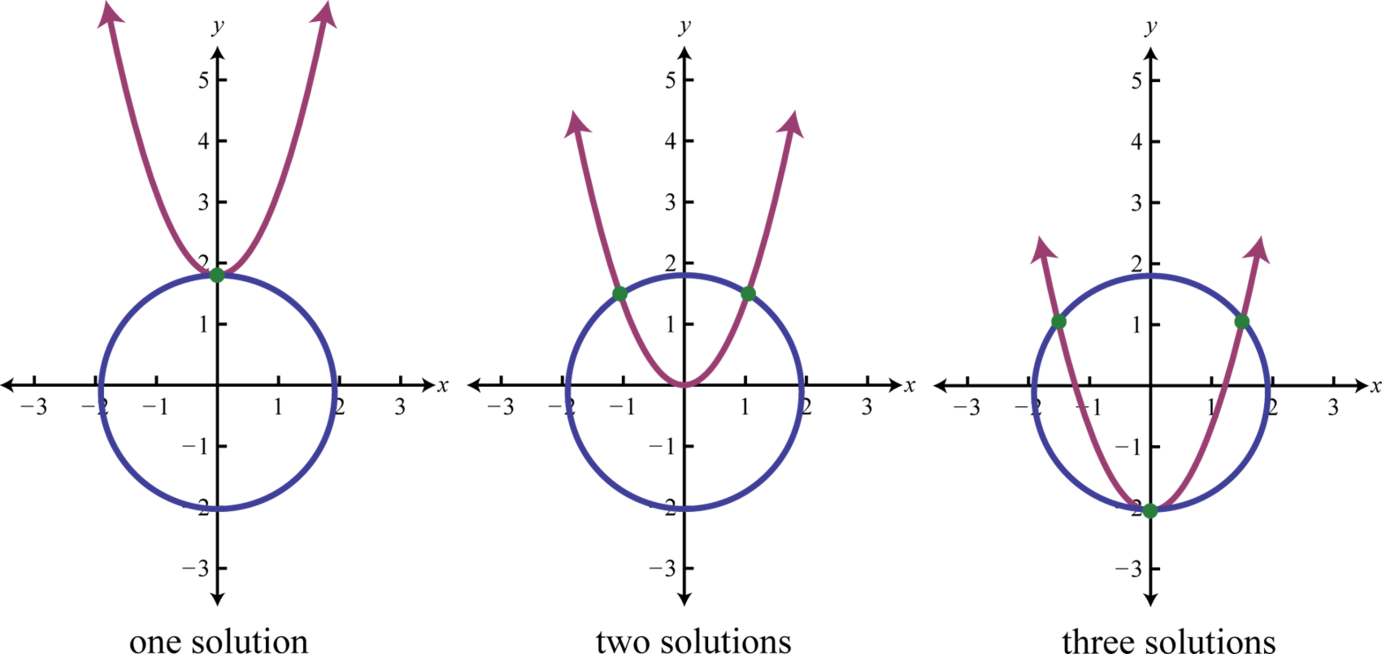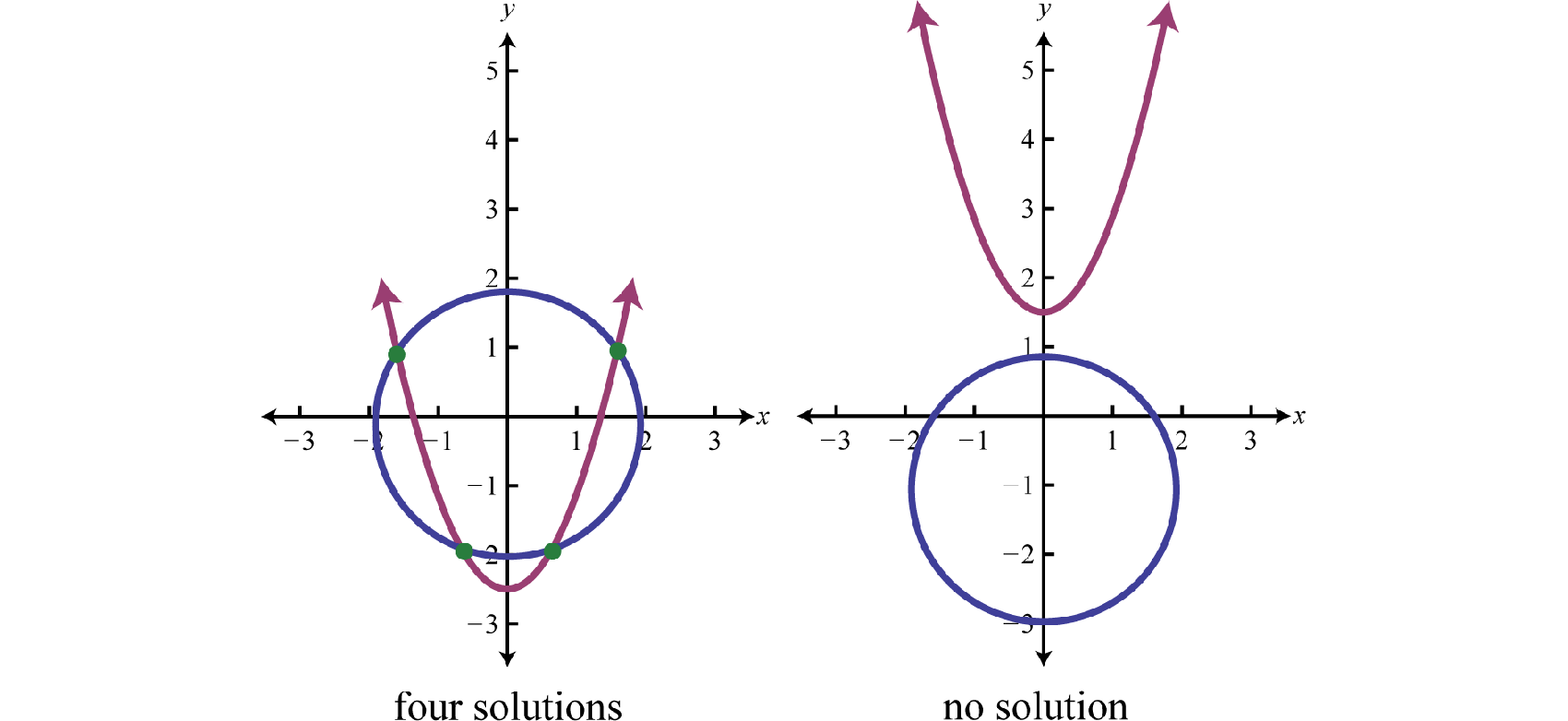This is “Solving Nonlinear Systems”, section 8.5 from the book Advanced Algebra (v. 1.0). For details on it (including licensing), click here.

Has this book helped you? Consider passing it on:
Creative Commons supports free culture from music to education. Their licenses helped make this book available to you.
DonorsChoose.org helps people like you help teachers fund their classroom projects, from art supplies to books to calculators.

## 8.5 Solving Nonlinear Systems

### Learning Objectives

1. Identify nonlinear systems.
2. Solve nonlinear systems using the substitution method.

## Nonlinear Systems

A system of equations where at least one equation is not linear is called a nonlinear systemA system of equations where at least one equation is not linear.. In this section we will use the substitution method to solve nonlinear systems. Recall that solutions to a system with two variables are ordered pairs $(x,y)$ that satisfy both equations.

### Example 1

Solve: ${x+2y=0x2+y2=5$.

Solution:

In this case we begin by solving for x in the first equation.

${x+2y=0 ⇒ x=−2yx2+y2=5$

Substitute $x=−2y$ into the second equation and then solve for y.

$(−2y)2+y2=54y2+y2=55y2=5y2=1y=±1$

Here there are two answers for y; use $x=−2y$ to find the corresponding x-values.

Using $y=−1$

Using $y=1$

$x=−2y=−2(−1)=2$

$x=−2y=−2(1)=−2$

This gives us two ordered pair solutions, $(2,−1)$ and $(−2,1).$

Answer: $(2,−1)$, $(−2,1)$

In the previous example, the given system consisted of a line and a circle. Graphing these equations on the same set of axes, we can see that the two ordered pair solutions correspond to the two points of intersection.If we are given a system consisting of a circle and a line, then there are 3 possibilities for real solutions—two solutions as pictured above, one solution, or no solution.### Example 2

Solve: ${x+y=3x2+y2=2$.

Solution:

Solve for y in the first equation.

${x+y=3 ⇒ y=3−xx2+y2=2$

Next, substitute $y=3−x$ into the second equation and then solve for x.

$x2+(3−x)2=2x2+9−6x+x2=22x2−6x+9=22x2−6x+7=0$

The resulting equation does not factor. Furthermore, using $a=2$, $b=−6$, and $c=7$ we can see that the discriminant is negative:

$b2−4ac=(−6)2−4(2)(7)=36−56=−20$

We conclude that there are no real solutions to this equation and thus no solution to the system.

Try this! Solve: ${x−y=5x2+(y+1)2=8$.

Answer: $(2,−3)$

If given a circle and a parabola, then there are 5 possibilities for solutions.When using the substitution method, we can perform the substitution step using entire algebraic expressions. The goal is to produce a single equation in one variable that can be solved using the techniques learned up to this point in our study of algebra.

### Example 3

Solve: ${x2+y2=2y−x2=−2$.

Solution:

We can solve for $x2$ in the second equation.

${x2+y2=2y−x2=−2 ⇒ y+2=x2$

Substitute $x2=y+2$ into the first equation and then solve for y.

$y+2+y2=2y2+y=0y(y+1)=0y=0 or y=−1$

Back substitute into $x2=y+2$ to find the corresponding x-values.

Using $y=−1$

Using $y=0$

$x2=y+2x2=−1+2x2=1x=±1$

$x2=y+2x2=0+2x2=2x=±2$

This leads us to four solutions, $(±1,−1)$ and $(±2,0).$

Answer: $(±1,−1)$, $(±2,0)$

### Example 4

Solve: ${(x−1)2−2y2=4x2+y2=9$.

Solution:

We can solve for $y2$ in the second equation,

${(x−1)2−2y2=4x2+y2=9 ⇒ y2=9−x2$

Substitute $y2=9−x2$ into the first equation and then solve for x.

$(x−1)2−2(9−x2)=4x2−2x+1−18+2x2=03x2−2x−21=0(3x+7)(x−3)=03x+7=0 or x−3=0x=−73x=3$

Back substitute into $y2=9−x2$ to find the corresponding y-values.

Using $x=−73$

Using $x=3$

$y2=9−(−73)2y2=91−499y2=329y=±323=±423$

$y2=9−(3)2y2=0y=0$

This leads to three solutions, $(−73,±423)$ and $(3,0).$

Answer: $(3,0)$, $(−73,±423)$

### Example 5

Solve: ${x2+y2=2xy=1$.

Solution:

Solve for $y$ in the second equation.

${x2+y2=2xy=1 ⇒ y=1x$

Substitute $y=1x$ into the first equation and then solve for x.

$x2+(1x)2=2x2+1x2=2$

This leaves us with a rational equation. Make a note that $x≠0$ and multiply both sides by $x2.$

$x2(x2+1x2)=2⋅x2x4+1=2x2x4−2x2+1=0(x2−1)(x2−1)=0$

At this point we can see that both factors are the same. Apply the zero product property.

$x2−1=0x2=1x=±1$

Back substitute into $y=1x$ to find the corresponding y-values.

Using $x=−1$

Using $x=1$

$y=1x=1−1=−1$

$y=1x=11=1$

Answer: $(1,1)$, $(−1,−1)$

Try this! Solve: ${1x+1y=41x2+1y2=40$.

Answer: $(−12,16)$, $(16,−12)$

### Key Takeaways

• Use the substitution method to solve nonlinear systems.
• Streamline the solving process by using entire algebraic expressions in the substitution step to obtain a single equation with one variable.
• Understanding the geometric interpretation of the system can help in finding real solutions.

### Part A: Nonlinear Systems

Solve.

1. ${x2+y2=10x+y=4$
2. ${x2+y2=5x−y=−3$
3. ${x2+y2=30x−3y=0$
4. ${x2+y2=102x−y=0$
5. ${x2+y2=182x−2y=−12$
6. ${(x−4)2+y2=254x−3y=16$
7. ${3x2+2y2=213x−y=0$
8. ${x2+5y2=36x−2y=0$
9. ${4x2+9y2=362x+3y=6$
10. ${4x2+y2=42x+y=−2$
11. ${2x2+y2=1x+y=1$
12. ${4x2+3y2=122x−y=2$
13. ${x2−2y2=35x−3y=0$
14. ${5x2−7y2=392x+4y=0$
15. ${9x2−4y2=363x+2y=0$
16. ${x2+y2=25x−2y=−12$
17. ${2x2+3y=98x−4y=12$
18. ${2x−4y2=33x−12y=6$
19. ${4x2+3y2=12x−32=0$
20. ${5x2+4y2=40y−3=0$
21. The sum of the squares of two positive integers is 10. If the first integer is added to twice the second integer, the sum is 7. Find the integers.

22. The diagonal of a rectangle measures $5$ units and has a perimeter equal to 6 units. Find the dimensions of the rectangle.

23. For what values of b will the following system have real solutions? ${x2+y2=1y=x+b$

24. For what values of m will the following system have real solutions? ${x2−y2=1y=mx$

Solve.

1. ${x2+y2=4y−x2=2$
2. ${x2+y2=4y−x2=−2$
3. ${x2+y2=4y−x2=3$
4. ${x2+y2=44y−x2=−4$
5. ${x2+3y2=9y2−x=3$
6. ${x2+3y2=9x+y2=−4$
7. ${4x2−3y2=12x2+y2=1$
8. ${x2+y2=1x2−y2=1$
9. ${x2+y2=14y2−x2−4y=0$
10. ${x2+y2=42x2−y2+4x=0$
11. ${2(x−2)2+y2=6(x−3)2+y2=4$
12. ${x2+y2−6y=04x2+5y2+20y=0$
13. ${x2+4y2=254x2+y2=40$
14. ${x2−2y2=−104x2+y2=10$
15. ${2x2+y2=14x2−(y−1)2=6$
16. ${3x2−(y−2)2=12x2+(y−2)2=1$
17. The difference of the squares of two positive integers is 12. The sum of the larger integer and the square of the smaller is equal to 8. Find the integers.

18. The difference between the length and width of a rectangle is 4 units and the diagonal measures 8 units. Find the dimensions of the rectangle. Round off to the nearest tenth.

19. The diagonal of a rectangle measures p units and has a perimeter equal to 2q units. Find the dimensions of the rectangle in terms of p and q.

20. The area of a rectangle is p square units and its perimeter is 2q units. Find the dimensions of the rectangle in terms of p and q.

Solve.

1. ${x2+y2=26xy=5$
2. ${x2+y2=10xy=3$
3. ${2x2−3y2=5xy=1$
4. ${3x2−4y2=−11xy=1$
5. ${x2+y2=2xy−2=0$
6. ${x2+y2=12xy−1=0$
7. ${4x−y2=0xy=2$
8. ${3y−x2=0xy−9=0$
9. ${2y−x2=0xy−1=0$
10. ${x−y2=0xy=3$
11. The diagonal of a rectangle measures $210$ units. If the area of the rectangle is 12 square units, find its dimensions.

12. The area of a rectangle is 48 square meters and the perimeter measures 32 meters. Find the dimensions of the rectangle.

13. The product of two positive integers is 72 and their sum is 18. Find the integers.

14. The sum of the squares of two positive integers is 52 and their product is 24. Find the integers.

Solve.

1. ${1x+1y=41x−1y=2$
2. ${2x−1y=51x+1y=2$
3. ${1x+2y=13x−1y=2$
4. ${1x+1y=61x2+1y2=20$
5. ${1x+1y=21x2+1y2=34$
6. ${xy−16=02x2−y=0$
7. ${x+y2=4y=x$
8. ${y2−(x−1)2=1y=x$
9. ${y=2xy=22x−56$
10. ${y=32x−72y−3x=0$
11. ${y=e4xy=e2x+6$
12. ${y−e2x=0y−ex=0$

### Part B: Discussion Board

1. How many real solutions can be obtained from a system that consists of a circle and a hyperbola? Explain.

2. Make up your own nonlinear system, solve it, and provide the answer. Also, provide a graph and discuss the geometric interpretation of the solutions.

1. $(1,3),(3,1)$

2. $(−33,−3),(33,3)$

3. $(−3,3)$

4. $(−1,−3)$, $(1,3)$

5. $(0,2)$, $(3,0)$

6. $(0,1)$, $(23,13)$

7. $(−35,−5)$, $(35,5)$

8. Ø

9. $(−3+352,−6+35)$, $(−3−352,−6−35)$

10. $(32,−1)$, $(32,1)$

11. 1, 3

12. $b∈[−2,2]$

13. $(0,2)$

14. Ø

15. $(−3,0)$, $(0,−3)$, $(0,3)$

16. Ø

17. $(0,1)$, $(−255,−15)$, $(255,−15)$

18. $(3,−2)$, $(3,2)$

19. $(−3,−2)$, $(−3,2)$, $(3,−2)$, $(3,2)$

20. $(−7,0)$, $(7,0)$, $(−553,43)$, $(553,43)$

21. 2, 4

22. $q+2p2−q22$ units by $q−2p2−q22$ units

23. $(−5,−1)$, $(5,1)$, $(−1,−5)$, $(1,5)$

24. $(−3,−33)$, $(3,33)$

25. Ø

26. $(1,2)$

27. $(23,432)$

28. 2 units by 6 units

29. 6, 12

30. $(13,1)$

31. $(75,7)$

32. $(−13,15)$, $(15,−13)$

33. $(2,2)$

34. $(3,8)$

35. $(ln 32,9)$